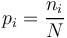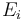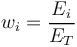# Concentration Index versus Diversity Index

## Concentration Index versus Diversity Index

Concentration indexes and diversity indexes are related families of metrics that aim to assess the prevalence of some category within a broader set.

## Discrete versus Continuous data sets

The main different between a concentration index and a diversity index is in the nature of the statistical observations that are used as input data for the index calculation. There are two possible starting points for input data:

## Examples

• Discrete: For example$p_i = \frac{n_i}{N}$ is the proportion of individuals in a population with total count N that belongs to Category i. A number of indexes used in diversity studies (Abundance, Richness, Margalev's, Menhinick’s diversity indexes) are based on such species counts.
• Numerical For example$E_i$ is a range of observations of some continuous quantity E such as risk Exposure or income. Such data will typically converted into weights$w_i = \frac{E_i}{E_T}$